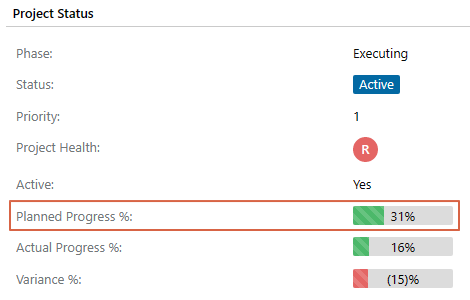Follow

# How does PPO calculate planned and actual progress percentages on a project level?

PPO calculates the overall planned and actual progress percentages of your project. These calculations provide an accurate, calculated view of the advancement of a project without relying on the project managers to import their schedules on a regular basis.

The below fields are calculated and can be seen on the Project entity: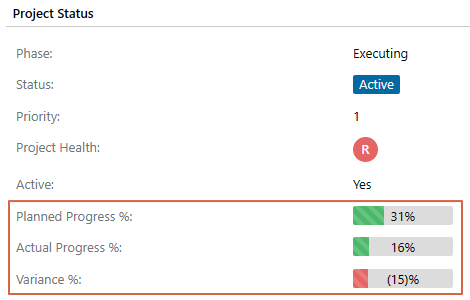How are these fields calculated?

Although PPO does these calculations, the calculation steps are explained here to provide the user with an understanding of which fields and values are used to determine the planned and actual progress percentages.

### ACTUAL PROGRESS PERCENTAGE CALCULATION

The Actual Progress % field is calculated as follows:

Step 1 - Determine the duration of the ENTIRE PROJECT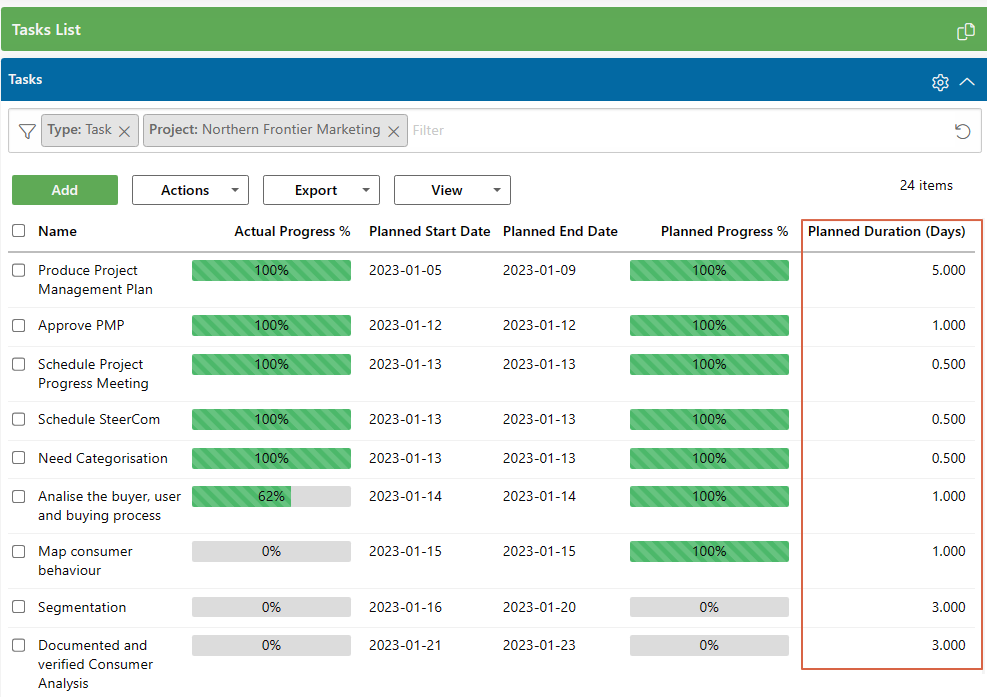In the example above, the total planned duration of the project is: 50.75 days.

Next, the Planned Duration of all the completed Tasks is added together.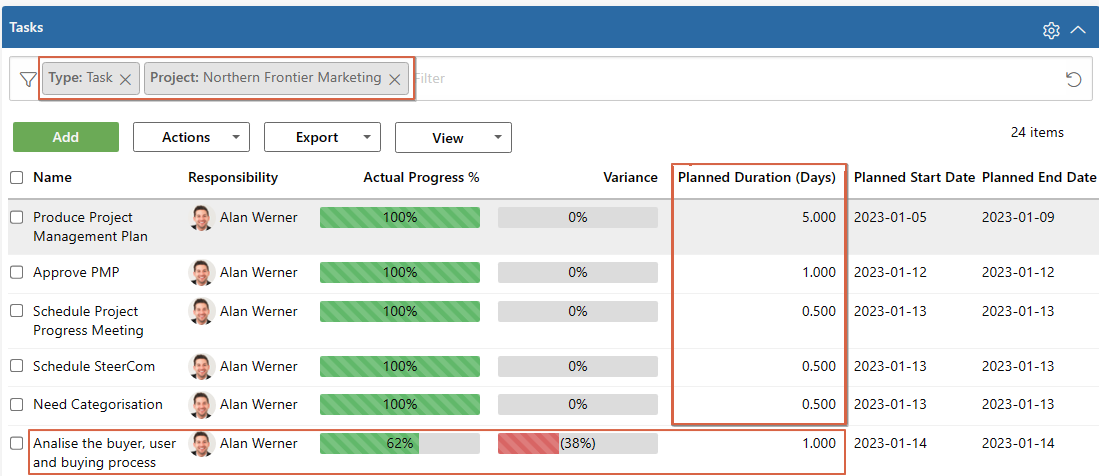In the example above, the Planned Duration of all the completed Tasks is 7.5 days.

However, there is also a Task that is partially completed (62 percent actual progress, marked in blue above). To determine the duration of the portion of the Task that is completed, the Actual Progress % is multiplied by the Planned Duration (62 percent x 1 day). The result is 0.62 days. Thus, we add the 0.62 days which have already been completed for this task to the duration of the completed Tasks.

The total number of days that have already been completed for this project is thus: 8.12 days.

Step 3 - Calculate the ACTUAL PROGRESS %

Lastly, to determine the percentage of work that has already been completed (Actual Progress %) the number of completed days is divided by the total number of days and shown as a percentage.

Therefore, (8.12 days / 50.75 days) x 100 percent = 16 percent

This is then reflected in the calculated field for the actual progress percentage on the Projects entity.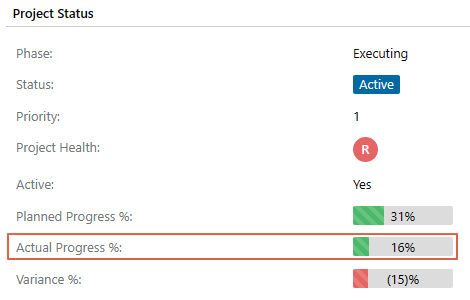### PLANNED PROGRESS PERCENTAGE

The Planned Progress % field is calculated as follows:

Step 1 - Determine the duration of the ENTIRE PROJECT

Determine the duration of the entire project as per Step 1 for the actual progress percentage. To recap this is done by adding up the Planned Duration field of all the tasks on the Tasks entity. This is only done for the tasks where the task type is "Task" (not summary tasks, milestones or the project task).

Step 2 - Determine the duration of TASKS UP TO TODAY

Next, calculate the duration of all Tasks that were planned to be completed by today.

For example, if today's date is10 April 2022, the Planned Duration of all the Tasks set to start and end before 10 April 2022 will be added up.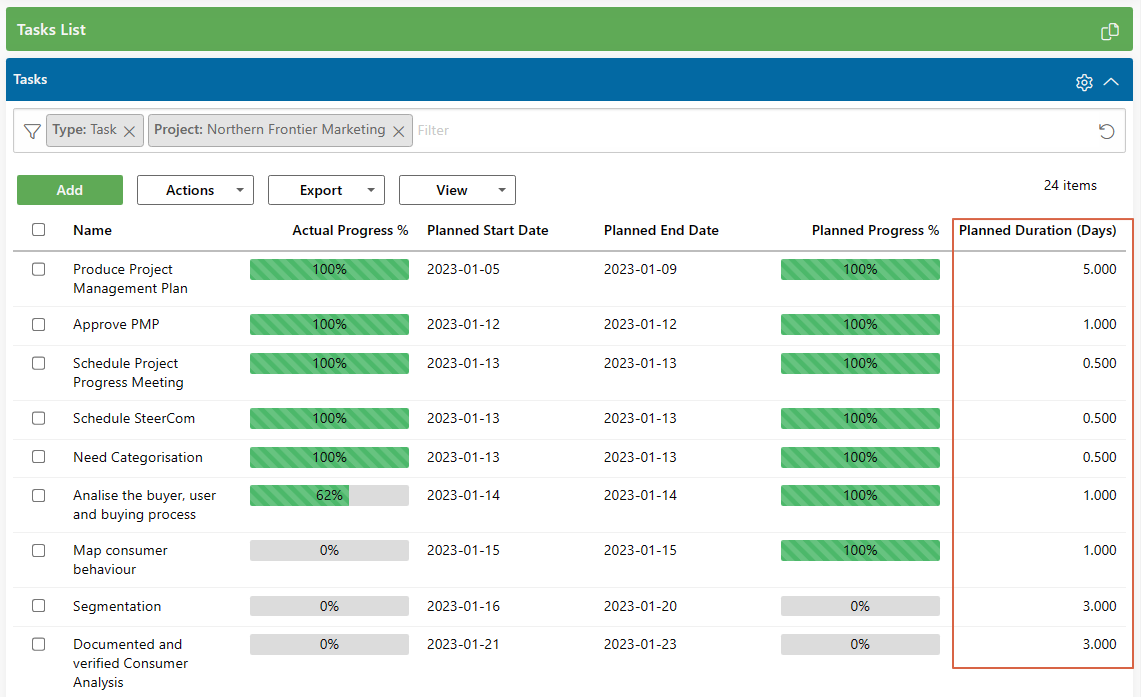In the example above, the Planned Duration of all the tasks planned to be completed by 2 April is 15.5 days.

Please note there could be tasks that could be partially completed by today. The task could have a Planned Duration of three days. By the end of today, a full day's work on the planned task should have been completed. When calculating the planned duration in days just keep this in mind.

Step 3 - Calculate the Planned Progress Percentage

Lastly, to determine the percentage of work that should have been completed by today (Planned Progress %) the number of completed days is divided by the total number of days and shown as a percentage.

Therefore, (15.5 days / 50.75 days) x 100 percent = 30.54 percent,which rounds up to 31 percent.

This is then reflected in the calculated field for Planned Progress % on the Projects entity.